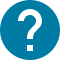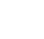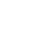大学院
HOME 大学院 情報と計測

# 情報と計測

The primary goal of the course is to provide students with an integrated view of how the basic concepts of mathematics and physics are applied to collecting information about a system. Upon successful completion of this course, the student will have:
-acquired a basic knowledge of the physics of information,
-gained an understanding of the physical principles of a broad range of modern sensors,
-developed skills in data analysis and system modeling.

コース名

3724-080
GEN-ME5601L3

ﾄﾞﾛﾈｰ ｼﾞｬﾝ ｼﾞｬｯｸ
S1

2

NO

Introduction to course Course goal and outline, class schedule, assignments and exams. Introduction to information theory and statistics -Introduction to information theory: information, binary choices, probability, entropy [bit], information transmission rate, sensor, calibration, signal vs. message, code, sampling, noise sources, effect of noise, channel capacity, noise can be helpful! (dithering) -Measurement errors and uncertainties: measurement, true value, random and systematic errors, uncertainty due to random errors, uncertainty due to systematic errors, total uncertainty, propagation of uncertainties, combination of separate measurements, correlation and regression -Examples and case studies: sensor fusion, rejection of data, error analysis in flow measurement (ASME standard on measurement uncertainty), stochastic resonance in natural systems Instrument and measurement -Sensor characteristics: transfer function, sensitivity, dynamic range, uncertainty, sensor classification -Dielectric materials for sensing: capacitive sensors, piezoelectric effect, piezoelectric crystal resonator -Optical fundamentals: wavefront, image quality (aberration, diffraction) -Optical microscopy: Kohler illumination, bright and dark fields, phase-shift microscopy (Zernike), differential interference contrast, fluorescence microscopy, confocal microscopy -Interferometry: Maxwell’s equations, interferometers (Michelson, Mach-Zender, Fabry-Perot), Fourier transform infrared spectrometer (case study), problems -Holography: scalar diffraction theory, wavefront reconstruction, in-line hologram (Gabor), off-axis hologram (Leith), Fourier hologram, applications -Light sensors: light interaction with matter, phototubes, photoresistors, p-n junction, p-i-n junction, metal-semiconductor junction -Magnetic sensors: spin, magnetic moment, diamagnetism, paramagnetism, ferromagnetism, Hall’s effect, magnetoresistance effects -Surface-plasmon-resonance based sensors: optical properties of metal, evanescent fields, surface plasmon resonance, bio-sensors Data analysis and modeling Signal processing: Fourier transform, sampling theorem, data pre-processing; Case study: lock-in amplification, zero padding Time series modeling: stationarity, autoregressive average models, nearest neighbor models, neural network models

1-hour written test　(open book)

J. R. Taylor, An introduction to error analysis, University Science Books, 1997. J. Fraden, Handbook of modern sensors, AIP press, Springer, 2003. R. Figliola, D. Beasley, Theory and design for mechanical measurements, Wiley, 2006.